By- Vineet Maheshwari
To infinity and beyond.

0 likes followers ViewsVineet Maheshwari

Followers
Views
0 Likes

# REGRESSION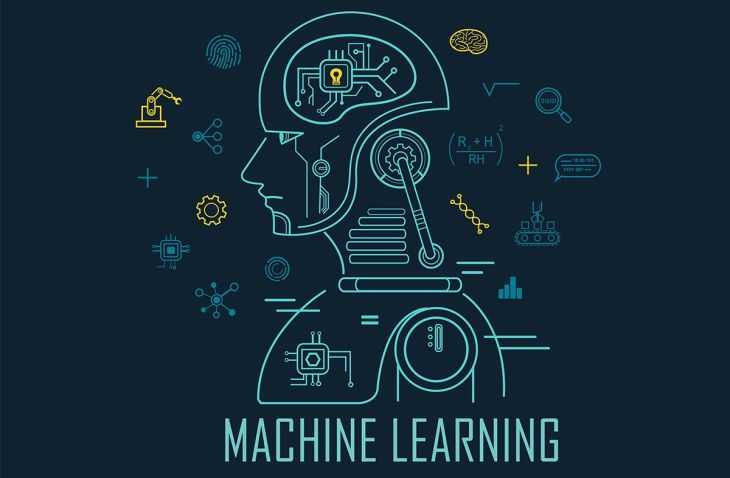Regression is a Machine Learning technique that falls under the category of Supervised ML technique. It is used to predict the output that is continuous not discrete. It analyzes the relationship between dependent and independent variable. It is often used for forecasting, time series modelling, e.t.c.

So basically Regression analysis is used for laying out the relationship between two kinds of variables, i.e dependent and independent and secondly, it is used for evaluating the impact of multiple independent variables on dependent variable.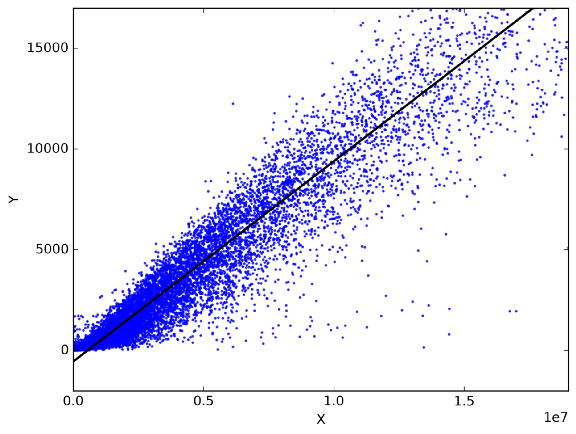There are different type of regression techniques:

1. Linear Regression
2. Logistic Regression
3. Polynomial Regression
4. Lasso Regression
5. Ridge Regression

Linear regression : It attempts to establish the relationship between two variables by fitting the linear equation on observed data. One variable is considered to be the independent variable or explanatory variable and the other one is known as dependent variable.

Y = a + bX

Here Y is the dependent variable, b is the slope of line that is being formed between variable Y and X, a is the intercept and X is the explanatory variable.

Dependent variable is always continuous, independent can be discrete or continuous. The nature of the line formed between these two variables is always Linear.

We have to keep in mind that we need to obtain the best fit line always, and this concept is being applied in every regression technique. This task is accomplished by Least Square Method, it is the well known method to fit the regression line.

Polynomial Regression : This is quite similar to Multiple Linear Regression, in this technique the relationship is being obtained by taking the k-th degree of variable X. Power of independent variable is more than 1.

Y = a + b * X^2

In this technique, the best fit line is a curve line that fits itself over the data points and this is the condition that differentiate it from linear regression, as in linear regression the best fit line is a Straight line.

While using this technique, we have to keep in mind that over-fitting and under-fitting does not take place. It should be the best fit.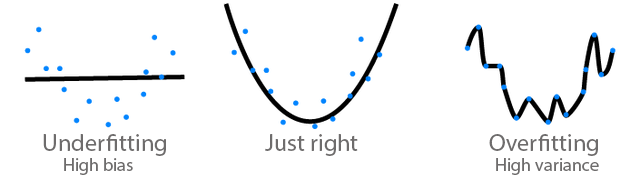Multiple Linear Regression : There was one explanatory variable in Linear technique, but this technique contains two or more explanatory variables.

As our independent variables are more than two, therefore we can use matrices more efficiently to define the regression model and doing subsequent analysis. In simple linear regression, error was being calculated at a fixed value of that single predictor, but in multiple linear, we have to find the error for a fixed set of values for all the predictors.

Here few hypothesis test are being conducted to check the values of different slope parameters that are involved in the formation of the equation and check the nature.

Implementation of Simple Linear Regression in python :

Now we will check the python implementation of Linear Regression model,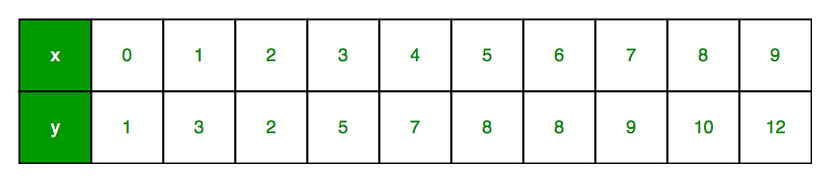source : geeksforgeeks.org

Here x is independent variable and y is a dependent variable or explanatory variable. And total 10 observations are there.

Below is the image of the scattered plot between these two variables. We have to work in such a way so that we can find the best fit line for this scattered plot, so that we can predict the most accurate results for new values.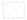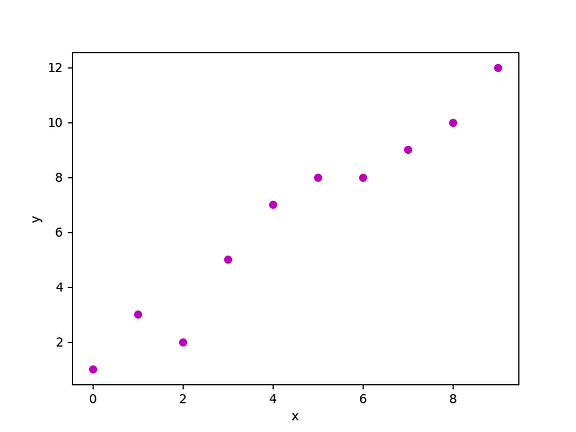source : geeksforgeeks.org

import pandas as pd

import numpy as np

import matplotlib.pyplot as plt

def estimate_coefficient(x,y):

n = np.size(x) #number of observations

mean_x = np.mean(x) #mean of x vector

mean_y = np.mean(y) #mean of y vector

cross = np.sum(y*x) - n*mean_x*mean_y

dev = np.sum(x*x) - n*mean_x*mean_x

#calculating regression coefficients

b = cross / dev

a = mean_y - b*mean_x

return(a,b)

def regression_line(x, y, b):

plt.scatter(x, y, color = "m", marker = "o", s = 30)

#now comes the predicted response vector

y_pred = a + b*x

#plotting regression line

plt.plot(x, y_pred, color = "g")

#labels

plt.xlabel('x')

plt.ylabel('y')

#function to show the graph

plt.show()

def main():

x = np.array([0, 1, 2, 3, 4, 5, 6, 7, 8, 9])

y = np.array([1, 3, 2, 5, 7, 8, 9, 10, 12])

#estimation of coefficients

b = estimate_coefficient(x,y)

#plot regression line

regression_line(x,y,b)

if __name__ == "__main__":

main()

Now the image or graph that will appear will look like this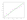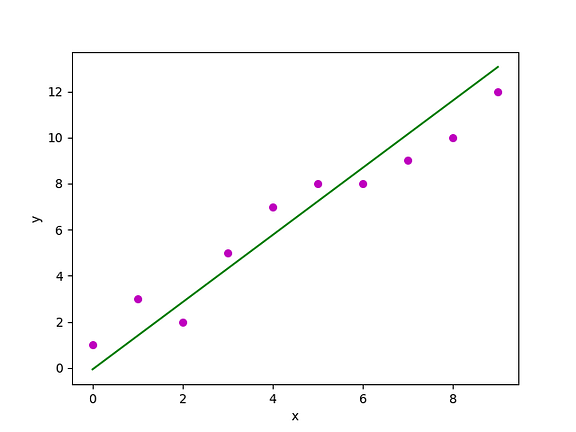source : geeksforgeeks.org

Now let’s check the Curve Fitting Process in Linear Regression :

Regression is all about fitting the model or curve on the data, so that we can predict the outputs for those points that are not being covered by the data. We have full information about data and model both, but we need to get the best fit model according to our data. In regression a lot of data is reduced into few parameters.

Curve fitting is the process to specify the model that provides the best fit to specific curves in our data set. Curved relationships between the variables are not that easy and straight to fit and interpret as linear relationships.

In linear relationships, if we change the value of independent variable by one unit, then the mean value of dependent variable also changes by some unit.

But in curved relationships, the change in dependent variable is not only dependent on the change in independent variable, rather it also depends on the location in the observation space. Therefore, effect of independent variable is, not a constant value.

Assumptions and conditions of linear regression :

There are few assumptions and conditions while building or working on Linear Regression model :

1. We keep on saying that the regression model is linear, linear regression and many more things, but here is one assumption to keep in mind that, the model is linear in terms of parameters.
2. We can apply regression model only on quantitative values, if our data is not the set of numbers than it is not advisable to apply regression.
3. Assigning numbers to categorical variables and then applying regression on them, it doesn’t work that way and results will not come as expected.
4. If our points are in a pattern, then obviously the values are being influenced by the errors, and what are errors, they are nothing but the deviation of observed value from the true value.
5. Homoscedasticity , when we want our points to look like a tube rather than a cone. Heteroscedasticity is like independence of error, where we see a trend in points, but here the trend is increasing or decreasing.

Applications of Regression :

1. It can be used in market research analysis.
2. It can be implemented as model for Predictive Analysis, for predicting the future opportunities.
3. Regression can be used to optimize the business process.
4. By reducing the tremendous amount of raw data into actionable information, regression analysis leads the way to smarter and more accurate decisions, and bring up some scientific angle to the management of the business.
5. And it is quite useful for identifying errors in judgement.